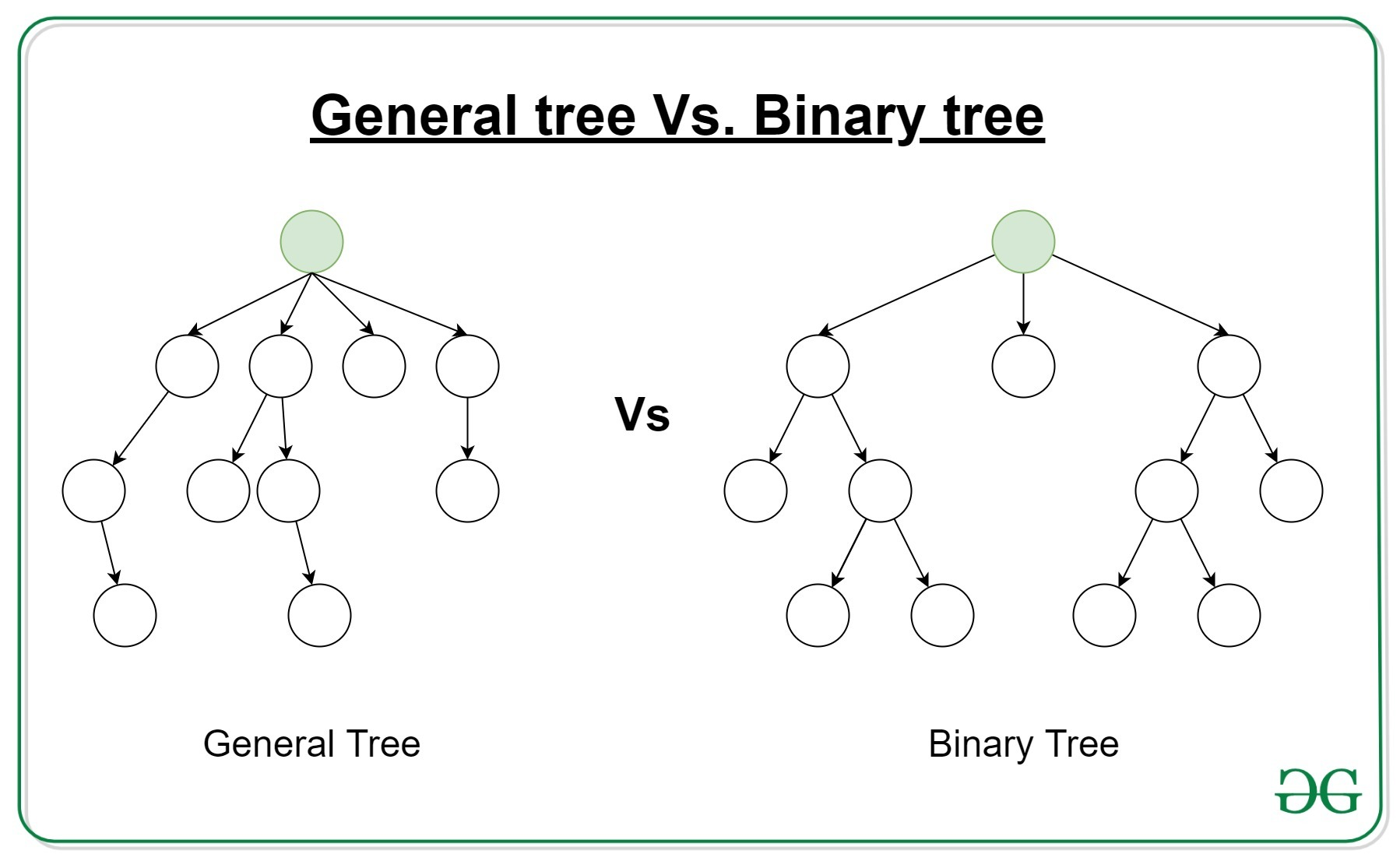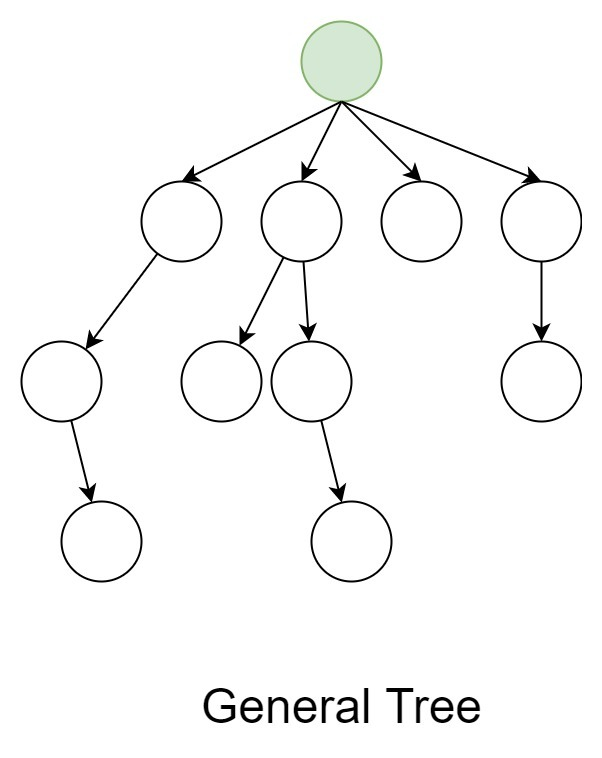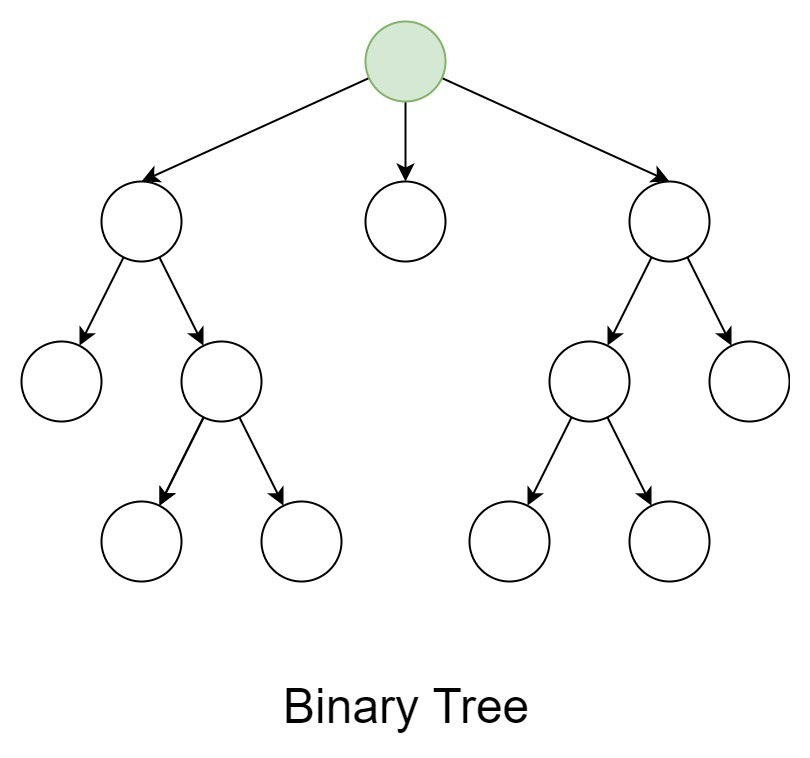# Difference between General tree and Binary treeGeneral Tree:
In the data structure, General tree is a tree in which each node can have either zero or many child nodes. It can not be empty. In general tree, there is no limitation on the degree of a node. The topmost node of a general tree is called the root node. There are many subtrees in a general tree. The subtree of a general tree is unordered because the nodes of the general tree can not be ordered according to specific criteria. In a general tree, each node has in-degree(number of parent nodes) one and maximum out-degree(number of child nodes) n.Binary Tree:
A binary tree is the specialized version of the General tree. A binary tree is a tree in which each node can have at most two nodes. In a binary tree, there is a limitation on the degree of a node because the nodes in a binary tree can’t have more than two child node(or degree two). The topmost node of a binary tree is called root node and there are mainly two subtrees one is left-subtree and another is right-subtree. Unlike the general tree, the binary tree can be empty. Unlike the general tree, the subtree of a binary tree is ordered because the nodes of a binary tree can be ordered according to specific criteria.Difference between General tree and Binary tree

General tree Binary tree
General tree is a tree in which each node can have many children or nodes. Whereas in binary tree, each node can have at most two nodes.
The subtree of a general tree do not hold the ordered property. While the subtree of binary tree hold the ordered property.
In data structure, a general tree can not be empty. While it can be empty.
In general tree, a node can have at most n(number of child nodes) nodes. While in binary tree, a node can have at most 2(number of child nodes) nodes.
In general tree, there is no limitation on the degree of a node. While in binary tree, there is limitation on the degree of a node because the nodes in a binary tree can’t have more than two child node.
In general tree, there is either zero subtree or many subtree. While in binary tree, there are mainly two subtree: Left-subtree and Right-subtree.

My Personal Notes arrow_drop_upCheck out this Author's contributed articles.

If you like GeeksforGeeks and would like to contribute, you can also write an article using contribute.geeksforgeeks.org or mail your article to contribute@geeksforgeeks.org. See your article appearing on the GeeksforGeeks main page and help other Geeks.

Please Improve this article if you find anything incorrect by clicking on the "Improve Article" button below.

Article Tags :
Practice Tags :

1

Please write to us at contribute@geeksforgeeks.org to report any issue with the above content.